Search IntMath
Close

# All About Lines in Geometry

In geometry, we use the term "line" to describe a straight path between two points. Both endpoints of the line extend to infinity.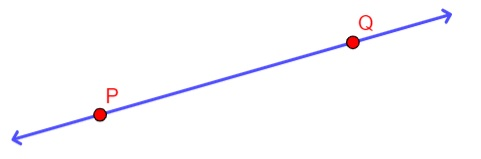The line PQ above and is represented by the notation: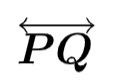# Horizontal, Vertical and Slant Lines

Lines can be classified on the basis of their alignment into the following:

• Horizontal Lines
• Vertical Lines
• Oblique or Slanting Lines

Horizontal lines are lines which are drawn from the left to the right without slanting. An example of a common horizontal line is the x-axis in the coordinate plane.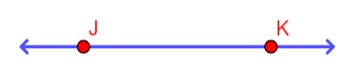Vertical lines are lines which are drawn from the bottom to the top without slanting. An example of a common vertical line is the y-axis in the coordinate plane.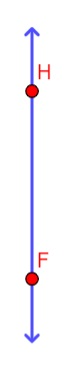If a line is neither vertical nor horizontal, then it is an oblique or slanting line.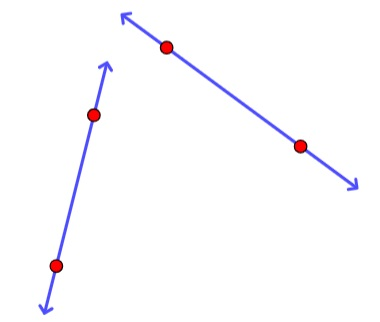# Intersecting or Non-intersecting Lines

Lines can be either intersecting or non-intersecting. Intersecting lines are lines that cross each other at some point, while non-intersecting lines never touch or cross. Let us take a closer look at both types of lines and how to identify them.

## Intersecting Lines

Intersecting lines are lines that cross each other at one point. To visualize this, think of the letter "X." These lines are characterized by the following properties:

• There is only one point of intersection between two lines.
• Intersecting lines form a pair of vertical angles at the point of intersection.
• The intersecting lines form a pair of vertical angles. The opposite angles of intersecting lines are equal while the adjacent angles form a linear pair.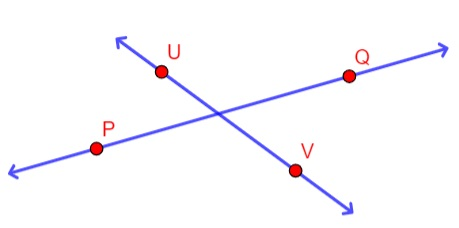When two lines intersect, they form four angles in two different ways.

## (I)Non-Perpendicular Intersecting Lines

Two of these angles are acute angles (less than 90 degrees), and two are obtuse angles (greater than 90 degrees). Here's an example of such intersecting lines: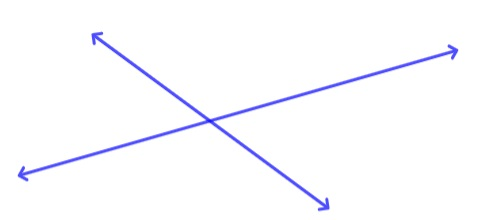Fig: Non perpendicular intersecting lines

## (II)Perpendicular Lines

If the angle between the two intersecting lines is 90 degrees, (i.e. a right angle), the two lines are said to be perpendicular. Here's an example of perpendicular lines.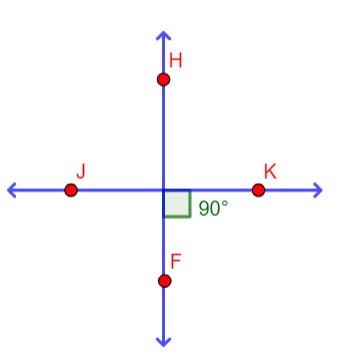Perpendicular intersecting lines

## Non-Intersecting Lines

Non-intersecting lines are lines that never touch or cross each other. These lines can be either parallel or skew.

Parallel lines are two lines that lie in the same plane and never intersect, no matter how far you extend them.

Skew lines on the other hand are lines that are in different planes, they are never parallel, and they never intersect. They occur in dimensions greater than 2-D.

For the purpose of this article, we will focus on parallel non-intersecting lines.

## Parallel Lines

Parallel lines are two lines that lie in the same plane and never intersect, no matter how far you extend them. They are characterized by the following properties:

• They never meet at any point.
• They have no point of intersection.
• The distance between any two points will always be the same.
• A line can have multiple non-intersecting lines.

Here's an example of non-intersecting, parallel lines: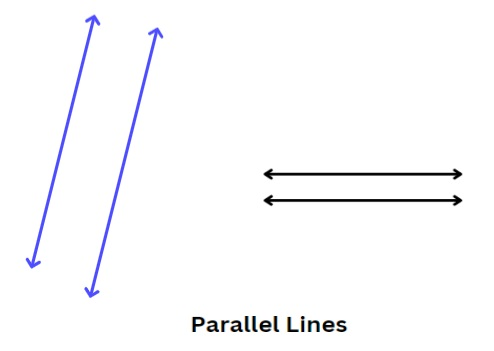## Other Terminologies

### Ray

A ray is a part of a line that has only one fixed point and the other point does not have any end. Examples of rays in our day-to-day lives are the sunlight or the light of a torch. The figure below represents the Ray JK.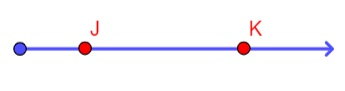Note that rays are labeled beginning with the point closest to the end. Ray JK is not the same as Ray KJ.

The notation for a ray is an arrow pointing towards one direction as shown below: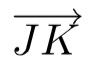### Segment

A segment is a part of a line that has a fixed length. Segments are sometimes also referred to as line segments. Examples of segments in our everyday lives are rulers, a pole, edge of a table etc.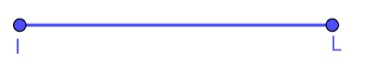A segment AB is represented by the notation## Conclusion:

Lines are a fundamental part of geometry. In this blog post, we learned about the two different types of lines: intersecting and non-intersecting. We also learned about parallel and perpendicular lines. In addition, the difference between lines, segments and rays was also stated.

Next time you're doing a geometry problem that involves lines, see if you can identify whether the lines are intersecting or non-intersecting and parallel or perpendicular.

## Problem SolverThis tool combines the power of mathematical computation engine that excels at solving mathematical formulas with the power of GPT large language models to parse and generate natural language. This creates math problem solver thats more accurate than ChatGPT, more flexible than a calculator, and faster answers than a human tutor. Learn More.